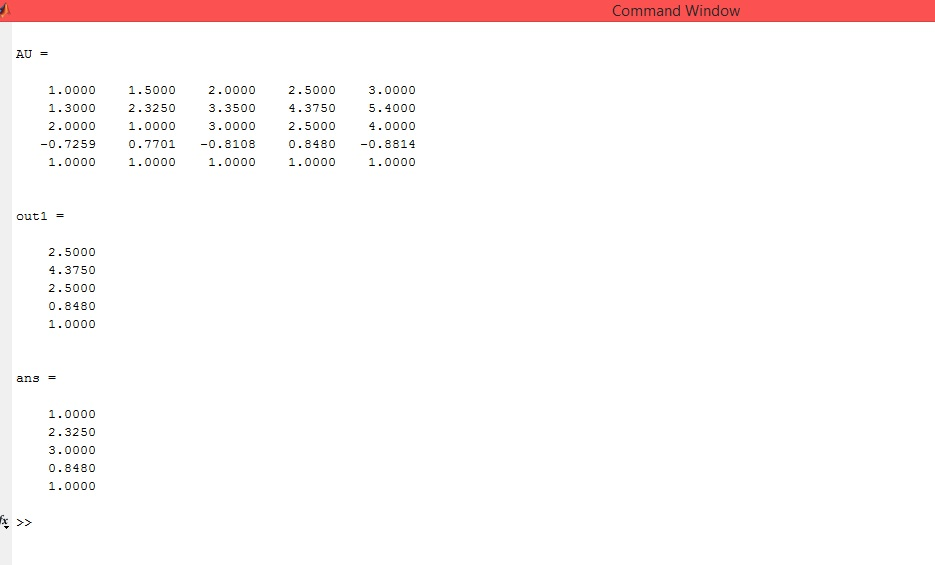# Answered! I need help with the following questions (blanks)… % Write commands to make sure all the variables in workspace are zero; _____??…

I need help with the following questions (blanks)…

% Write commands to make sure all the variables in workspace are zero; _____??

Don't use plagiarized sources. Get Your Custom Essay on
Answered! I need help with the following questions (blanks)… % Write commands to make sure all the variables in workspace are zero; _____??…
GET AN ESSAY WRITTEN FOR YOU FROM AS LOW AS \$13/PAGE

% also everything on screen is clean clc % Define the following arrays % a, that includes from 1 to 3 with increments 0.5 (use m:q:n format). a=[1:0.5:3]

% b, that includes 5 numbers from 1.3 to 5.4 with equal increments (use linspace) b=linspace(1.3,5.4,5)

% c, that includes 2, 1, 3, 2.5, and 4. c=[2 1 3 2.5 4]

% d, which is cos(3b) d=cos(3*b)

% e, which has 5 elements all are 1 (use “one” command) e=linspace(1,1,5) ??

% For all above arrays: values should not be shown on the screen, ____??

% All above arrays must be in form of row vectors

% Define a matrix, AU, that includes a, b, c, d, and e. ___ ??

% Define an array, out1, that includes all the values in column 4 of AU ( use M(:,n) ). ___ ??

% Use a MATLAB command to show all the diagonal values of matric AU. ___ ??

MATLAB CODE

clear all % command to make sure all the variables in workspace are zero

clc % Command to clean everything on screen

a = [1:0.5:3]; % vector a

b = linspace(1.3, 5.4, 5); % vector b

c = [2 1 3 2.5 4]; % vector c

d = cos(3*b); % row vector d

e = ones(1, 5); % vector of 1’s

% For all above arrays: values should not be shown on the screen, => append a semicolon ( ; ) after each statement (already appended)

AU = [a; b; c; d; e] % Matrix AU

out1 = AU(:, 4) % extracting 4th column of matrix AU

diag(AU) % Displaying diagonal of matrix AU

OUTPUT